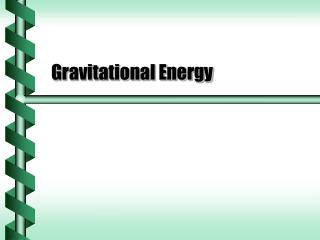Download PresentationGravitational Energy

Loading in 2 Seconds...

# Gravitational Energy - PowerPoint PPT Presentation

Gravitational Energy. Gravitational Work. Gravity on the surface of the Earth is a local consequence of universal gravitation. How much work can an object falling from very far from the Earth do when it hits the surface?. r. R E. Gravitational Potential. The work doesn’t depend on the path.I am the owner, or an agent authorized to act on behalf of the owner, of the copyrighted work described.
Download Presentation## Gravitational Energy

An Image/Link below is provided (as is) to download presentation

Download Policy: Content on the Website is provided to you AS IS for your information and personal use and may not be sold / licensed / shared on other websites without getting consent from its author.While downloading, if for some reason you are not able to download a presentation, the publisher may have deleted the file from their server.

- - - - - - - - - - - - - - - - - - - - - - - - - - E N D - - - - - - - - - - - - - - - - - - - - - - - - - -
Presentation Transcript
1. Gravitational Energy

2. Gravitational Work • Gravity on the surface of the Earth is a local consequence of universal gravitation. • How much work can an object falling from very far from the Earth do when it hits the surface? r RE

3. Gravitational Potential • The work doesn’t depend on the path. • Universal gravity is a conservative force • The potential is set with U = 0 at an infinite distance. • Gravity acts at all ranges • Gravity is weakest far from the source

4. For circular motion there speed is related to the centripetal acceleration. a = v2/r An object moving in a circle has kinetic energy. K = ½mv2 K = ½ mar K = ½ F r The kinetic energy is equal to half the work that could be done. Kinetic Energy in Circles v F r

5. The kinetic energy for a circular orbit is related to the potential energy. The total energy in a circular orbit can be described in terms of either the kinetic or the potential energy. Kinetic Energy in Orbit

6. Escape Velocity • Negative total energy can be viewed as being captured by the force of gravity. • To get away from the influence of gravity requires zero or positive energy. • The minimum velocity is called the escape velocity. On earth,vesc= 11.2 km/s next